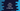# Java program to check if a year is a leap year or not## Java program to check if a year is a leap year or not:

In this Java programming tutorial, we will learn how to check if a year is leap year or not. This program will take one number as the input and print a message that it is leap year or it is not.

A year is a leap year if it is divisible by 4 and for century years, it is also divisible by 400. So, we need to check if the year is divisible by 4 and for century years, it is divisible by 400 or not.

Let’s understand the algorithm before start writing the program.

### Algorithm to use:

We will use the below algorithm:

• Take the year as input from the user.
• Check if the number is divisible by 4 or not. If not, then return false or it is not a leap year.
• If it is divisible by 4, we need to check if it is a century year or not. If it is divisible by 100, it is a century year.

• If it is not a century year, it is a leap year.
• If it is a century year, check if it is divisible by 400 or not. If yes, it is a leap year. Else, it is not.

Following years are leap year: 1904, 1908, 1912, 1916, 1920, 1924, 1928, 1932, 1936, 1940, 1944, 1948, 1952, 1956, 1960, 1964, 1968, 1972, 1976, 1980, 1984, 1988, 1992, 1996, 2000, 2004, 2008, 2012, 2016, 2020.

Let’s write it in code:

### Java program to check if a year is leap year or not:

Below program checks if a year is leap year or not:

``````import java.util.Scanner;

class Main {

public static boolean isLeapYear(int year) {
if (year % 4 == 0) {
if (year % 100 == 0) {
return year % 400 == 0;
} else {
return true;
}
}

return false;
}

public static void main(String[] args) {
int year;

Scanner sc = new Scanner(System.in);
System.out.println("Enter the number: ");
year = sc.nextInt();

if (isLeapYear(year)) {
System.out.println(year + " is a leap year");
} else {
System.out.println(year + " is not a leap year");
}

}
}``````

Here,

• isLeapYear method is used to check if a year is leap year or not. It takes the year as the parameter and returns one boolean value.
• The first if block checks if the value is divisible by 4 or not. If yes, it checks if it is divisible by 100 or not. If yes, it checks if it is divisible by 400. If not, it returns true.
• It returns false if the year value is not divisible by 4.

If you run this program, it will print output as like below:

``````Enter the number:
1988
1988 is a leap year

Enter the number:
1989
1989 is not a leap year``````

We can use a loop and use the method used in the above program to find out all leap years in a specific range. For example:

``````class Main {

public static boolean isLeapYear(int year) {
if (year % 4 == 0) {
if (year % 100 == 0) {
return year % 400 == 0;
} else {
return true;
}
}

return false;
}

public static void main(String[] args) {
for (int i = 1900; i < 2021; i++) {
if (isLeapYear(i)) {
System.out.println(i);
}
}

}
}``````

In this program, the for loop runs from 1900 to 2020 and it will print all leap years in between these two years.

``````1904
1908
1912
1916
1920
1924
1928
1932
1936
1940
1944
1948
1952
1956
1960
1964
1968
1972
1976
1980
1984
1988
1992
1996
2000
2004
2008
2012
2016
2020``````